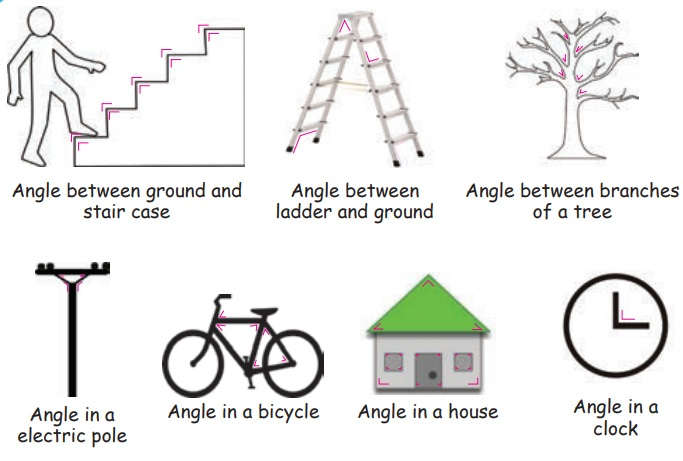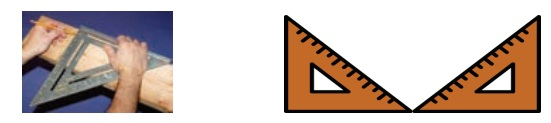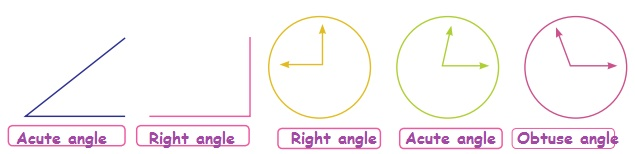Home | | Maths 5th Std | Introduction of Angles

# Introduction of Angles

a. To get the feel of an angle through observation of objects and by paper folding: b. Able to learn the names of angle like acute, obtuse and right angle: c. Able to identify right angles in the environment:

Introduction of Angles

a. To get the feel of an angle through observation of objects and by paper folding:

Bridges, buildings, cell phone towers, wings of planes, bicycles, windows doors and things around us have angle in them.Angle:

The figure formed by two rays/ with a common point is called an angle.Teacher: What does this the picture show?

Ramu: This picture shows an angle. Does angle have name sir?Teacher: Yes. Angles have names. Can you see two line segments in this picture? Read the names of that.

Ramu: Sir, This angle has two line segments. They are BA and BC.

Teacher: Which is the common point of the two line segments?

Ramu: B is the common point.

Teacher: These two line segments make an angle. Common point B is vertex. BA&BC are arms of the angle.

Ramu : Then how can we name the angle in the picture?

Teacher: An angle is mentioned by three alphabet. The centre letter of the angle denotes the vertex.

Ramu: Then, ABC is the name of the angle. Am I right sir?

Teacher: Yes, We should mention the angle as angle ABC.

Ramu: Sir, can we write angle CBA instead of angle ABC.

Teacher: Surely. Angle ABC and Angle CBA are same. We use this symbol for angle.

So we can write angle ABC as ABC.Angle between ground and stair case

Angle between branches of a tree

Angle in a electric pole

Angle in a bicycle

Angle in a house

Angle in a Clock

Find:

In this picture, mark the angles formed inside and outside by using colour pencils.Project:

Collect some pictures having angles, and paste the same in a chart.

Draw some angles by using pencil and show it to your teacher.Activity

Look at the angles formed by your elbow and draw them as stick picture and show it to your teacher. Please share it with your friends and check.

Word and meaning:

The word angle came from the Greek word “Angilos”. It means curved and not straight. Ankle is the place where knee and leg foot is joined.

b. Able to learn the names of angle like acute, obtuse and right angle:

We can create the various angles by combining the two wooden frames. Let us see the picture given below, from these shape we can find the types of angles.Write the angles generated in the following items. (Obtuse angle, acute angle, right angle)Try thisc. Able to identify right angles in the environment:

Ram is trying to cut a rectangular piece of wood, from a wooden board. Ram used a tool to cut sides of the rectangle. We call this device as set square.

We can see two set squares in a Geometry Box. It has 90° each.Examples of right angles:Do Yourself: Draw 5 objects with right angle.Classify the angles as acute, obtuse and right angle.Try these

Classify the following angles

30°, 45°, 60°, 90°, 120°, 130°, 170°, 75°

Acute angle: 30°, 45°, 60°, 75°

Right angle: 90°

Obtuse angle: 120°, 130°, 170°

Observe the following pictures and write the name of the angles in their box.Activity 1

Draw right angle, acute angle and obtuse angle by tracing.Project (Art and Craft)

1. Form the angles acute/obtuse/right angle by cutting/folding the papers and paste it in a chart.2. Write the names of the flowers or animals (or) birds in English capital letter and mention the angles in it.Tags : Geometry | Term 1 Chapter 1 | 5th Maths , 5th Maths : Term 1 Unit 1 : Geometry
Study Material, Lecturing Notes, Assignment, Reference, Wiki description explanation, brief detail
5th Maths : Term 1 Unit 1 : Geometry : Introduction of Angles | Geometry | Term 1 Chapter 1 | 5th Maths